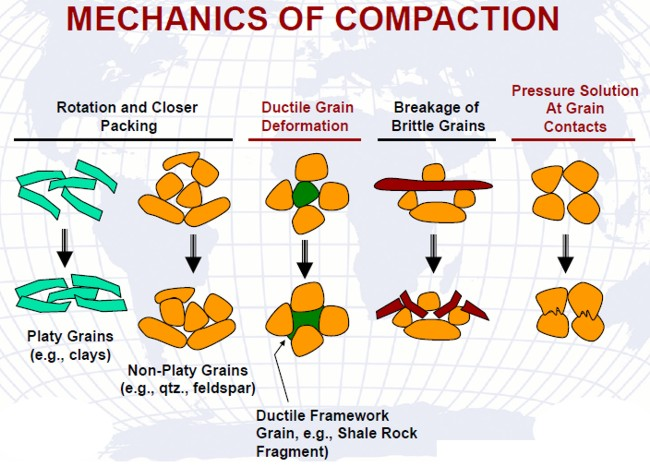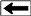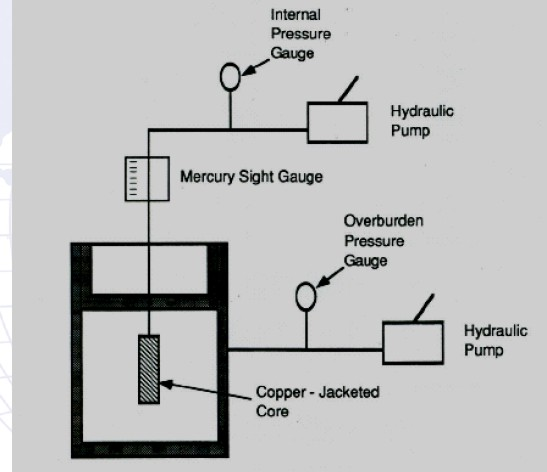ROCK COMPRESSIBILITY BASICS
The total pressure at any reservoir depth, due to the weight of overlying fluid saturated rock column, is called the overburden pressure, Po. The total pressure at any depth is the sum of the overlaying fluid-column pressure Pp and the overlaying grain or matrix column pressure Pm:
1: Po = Pp + Pm

A typical value of overburden pressure gradient is approximately one psi per foot of depth. Overburden pressure depends on depth, structure, consolidation of the formation, geologic age, and history of the rock.

The weight of the overburden applies a compressive force to the reservoir. The pressure in the rock pore spaces does not normally approach the overburden pressure. A typical pore pressure gradient, commonly referred to as the reservoir pressure, is approximately 0.45 psi per foot of depth, assuming that the reservoir is sufficiently consolidated so the overburden pressure is not transmitted to the fluids in the pore spaces. Some reservoirs are overpressured, with gradients as high as 1.0 psi/ft, and some are underpressured due to production of fluids.Effects of compression on rocks

Normal sedimentary processes of compaction compress the rocks, reducing porosity and sometimes changing the shape and size of rock grains, as shown in the illustration above.

The pressure difference between overburden and internal pore pressure is referred to as the effective overburden pressure. During pressure depletion (oil or gas production) operations, the internal pore pressure decreases and, therefore, the effective overburden pressure increases. This increase causes the bulk volume of the reservoir rock to reduce, and the rock grains to expand, reducing porosity.

Compressibility is the relative volume change of matter per unit pressure change under conditions of constant temperature. Usually, petroleum reservoirs can be considered isothermal (an exception: thermal stimulation such as steam assisted gravity drainage, SAGD, or fire floods). Increasing pressure causes volume of material to decrease (compression). Decreasing pressure causes volume expansion.

The coefficient of isothermal compressibility, C is always a positive value, which accounts for the negative sign in the following equations:
2: Cm = - (1 / Vm) * (dVm / dP)
3: Cp = - (1 / Vp) * (dVp / dP)
4: Cb = - (1 / Vb) * (dVb / dP)

Wjere:
Cm, Cp, Cb = rock matrix, pore space, and bulk compressibility (psi-1)
Vm, Vp, Vb = rock matrix, pore space, and bulk volume (cu.ft)
Pp = pore pressure (psi)
P =  effective pressure (psi)

For some oilfield purposes, Cm and Cb are small, and the composite formation compressibility Ct is assumed to be equal to Cp. Typical values for Ct are 3 to 25 * 10^-6 psi-1. Ct varies inversely with porosity and pressure, and numerous authors have published correlations applicable to specific rock types.

The inverse of a compressibility is a bulk modulus, for example Kc = 1 / Ct is the composite bulk modulus of the porous rock. Descriptive terms and  abbreviations used in the literature vary widely, for example Cr is sometimes used to represent the rock matrix compressibility Cm, and the rock matrix is sometimes referred to as the "empty rock frame".MEASURING COMPRESSIBILITY
In reservoirs, overburden pressure is constant and the pressure of fluid in pores changes, resulting in pore volume change. In the laboratory, we change the confining pressure on the core plug (overburden) while holding the pore pressure constant. The net compaction pressure on the matrix is the difference between the overburden and pore pressures, so the net effect is the same in both cases. This allows us to obtain useful results in the laboratory.

The laboratory procedure:  Core plug is 100% saturated with brine. Core plug is placed in rubber or soft copper sleeve. As pressure outside sleeve is increased, pore volume decreases and the volume of expelled brine is measured.Laboratory apparatus for measuring rock compressibilityELASTIC PROPERTIES FROM MICRO CT SCANSProcessing of micro CT scans to determine elastic moduli is done by by simulating a static deformation experiment on a 3D digital rock sample. These tests are nondestructive and can be run on small samples such as drill cuttings.

The application of stresses to the faces of the sample generates strains in the rock frame that are computed locally using the finite element method (FEM). The resulting effective deformations of the sample are related to the stresses applied at the boundaries to calculate the effective elastic moduli. This application assumes linear elasticity laws are valid within the sample. Therefore, the elastic moduli can be converted into the elastic-wave velocities.

Various loading configurations are applied to the same digital sample to obtain the effective elastic moduli (e.g., the bulk and shear). Source: www.ingrainrocks.com.Page Views ---- Since 01 Jan 2015
Copyright 2023 by Accessible Petrophysics Ltd.
CPH Logo, "CPH", "CPH Gold Member", "CPH Platinum Member", "Crain's Rules", "Meta/Log", "Computer-Ready-Math", "Petro/Fusion Scripts" are Trademarks of the Author